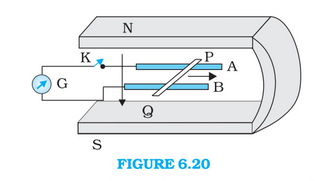Q

# In the Figure shows a metal rod PQ resting on the smooth rails AB and positioned between the poles of a permanent magnet. How much power is dissipated as heat in the closed circuit?

Q6.14 (f) Figure 6.20 shows a metal rod   resting on the smooth rails  and positioned between the poles of a permanent magnet. The rails, the rod, and the magnetic field are in three mutual perpendicular directions. A galvanometer  connects the rails through a switch . Length of the ,, resistance of the closed loop containing the . Assume the field to be uniform. Suppose K is open and the rod is moved with a speed of  in the direction shown. Give the polarity and magnitude of the induced emf

(f) How much power is dissipated as heat in the closed circuit? What is the source of this power?Views

Current in the circuit  = 1A

The resistance of the circuit  = 9m

The power which is dissipated as the heat

Hence 9mW of heat is dissipated.

We are moving the rod which induces the current. The external agent through which we are moving our rod is the source of the power.

Exams
Articles
Questions